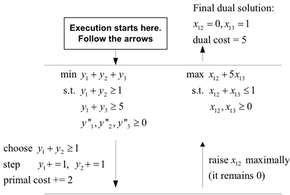## neal young / Koufogiannakis11Distributed

•This paper gives poly-logarithmic-round, distributed δ-approximation algorithms for covering problems with submodular cost and monotone covering constraints (Submodular-cost Covering). The approximation ratio δ is the maximum number of variables in any constraint. Special cases include Covering Mixed Integer Linear Programs (CMIP), and Weighted Vertex Cover (with δ = 2).

Via duality, the paper also gives poly-logarithmic-round, distributed δ-approximation algorithms for Fractional Packing linear programs (where δ is the maximum number of constraints in which any variable occurs), and for Max Weighted c-Matching in hypergraphs (where δ is the maximum size of any of the hyperedges; for graphs δ = 2).

The paper also gives parallel (RNC) 2-approximation algorithms for CMIP with two variables per constraint and Weighted Vertex Cover.

The algorithms are randomized. All of the approximation ratios exactly match those of comparable centralized algorithms.

(This paper gives distributed implementations of algorithms from Greedy δ-approximation algorithm for covering with arbitrary constraints and submodular cost .)
Journal version of two conference papers, on [covering] and [packing].